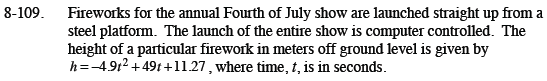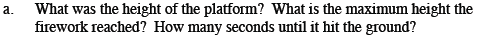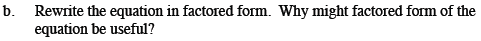Home > CCA2 > Chapter 8 > Lesson 8.2.3 > Problem8-109

8-109.
1. Fireworks for the annual Fourth of July show are launched straight up from a steel platform. The launch of the entire show is computer controlled. The height of a particular firework in meters off ground level is given by h = −4.9t2 + 49t +11.27, where time, t, is in seconds. Homework Help ✎

1. What was the height of the platform? What is the maximum height the firework reached? How many seconds until it hit the ground?

2. Rewrite the equation in factored form. Why might factored form of the equation be useful?Substitute t = 0 to find the height of the platform.

Use the Quadratic Formula to find the roots. Average them to find the line of symmetry.

You have now found the parameter h in the general equation y = a(xh)² + k.
The given equation has the same a value, −4.9.
Solve for k by substituting the point you found in part (a).

h(x) = -4.9(t − 5)² + 133.77The factored form reveals the intercepts. Remember to include the a value in your factored equation.